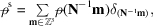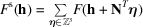International
Tables for
Crystallography
Volume B
Reciprocal space
Edited by U. Shmueli

International Tables for Crystallography (2010). Vol. B, ch. 1.3, pp. 93-94   | 1 | 2 |

## Section 1.3.4.4.5. Structure factors via model electron-density maps

G. Bricognea

aGlobal Phasing Ltd, Sheraton House, Suites 14–16, Castle Park, Cambridge CB3 0AX, England, and LURE, Bâtiment 209D, Université Paris-Sud, 91405 Orsay, France

#### 1.3.4.4.5. Structure factors via model electron-density maps

| top | pdf |

Robertson (1936b)recognized the similarity between the calculation of structure factors by Fourier summation and the calculation of Fourier syntheses, the main difference being of course that atomic coordinates do not usually lie exactly on a grid obtained by integer subdivision of the crystal lattice. He proposed to address this difficulty by the use of his sorting board, which could extend the scale of subdivision and thus avoid phase errors. In this way the calculation of structure factors became amenable to Beevers–Lipson strip methods, with considerable gain of speed.

Later, Beevers & Lipson (1952)proposed that trigonometric functions attached to atomic positions falling between the grid points on which Beevers–Lipson strips were based should be obtained by linear interpolation from the values found on the strips for the closest grid points. This amounts (Section 1.3.4.4.3.4) to using atoms in the shape of a trilinear wedge, whose form factor was indicated in Section 1.3.4.4.3.4and gives rise to aliasing effects (see below) not considered by Beevers & Lipson.

The correct formulation of this idea came with the work of Sayre (1951), who showed that structure factors could be calculated by Fourier analysis of a sampled electron-density map previously generated on a subdivisionof the crystal lattice Λ. When generating such a map, care must be taken to distribute onto the sample grid not only the electron densities of all the atoms in the asymmetric motif, but also those of their images under space-group symmetries and lattice translations. Considerable savings in computation occur, especially for large structures, because atoms are localized: each atom sends contributions to only a few grid points in real space, rather than to all reciprocal-lattice points. The generation of the sampled electron-density map is still of complexityfor N atoms andreflections, but the proportionality constant is smaller than that in Section 1.3.4.4.4by orders of magnitude; the extra cost of Fourier analysis, proportional to, is negligible.

The idea of approximating a Fourier transform by a discrete transform on sampled values had already been used by Whittaker (1948), who tested it on the first three odd Hermite functions and did not consider the problem of aliasing errors. By contrast, Sayre gave a lucid analysis of the sampling problems associated to this technique. If the periodic sampled map is written in the form of a weighted lattice distribution (as in Section 1.3.2.7.3) asthen its discrete Fourier transform yieldsso that each correct valueis corrupted by its aliasesfor.

To cure this aliasing problem, Sayre used hypothetical atoms' with form factors equal to those of standard atoms within the resolution range of interest, but set to zero outside that range. This amounts to using atomic densities with built-in series-termination errors, which has the detrimental effect of introducing slowly decaying ripples around the atom which require incrementing sample densities at many more grid points per atom.

Sayre considered another cure in the form of an artificial temperature factor B (Bragg & West, 1930) applied to all atoms. This spreads each atom on more grid points in real space but speeds up the decay of its transform in reciprocal space, thus allowing the use of a coarser sampling grid in real space. He discounted it as spoiling the agreement with observed data, but Ten Eyck (1977)pointed out that this agreement could be restored by applying the negative of the artificial temperature factor to the results. This idea cannot be carried to extremes: if B is chosen too large, the atoms will be so spread out in real space as each to occupy a sizeable fraction of the unit cell and the advantage of atom localization will be lost; furthermore, the form factors will fall off so rapidly that round-off error amplification will occur when the results are sharpened back. Clearly, there exists an optimal combination of B and sampling rate yielding the most economical computation for a given accuracy at a given resolution, and a formula will now be given to calculate it.

Let us make the simplifying assumption that all atoms are roughly equal and that their common form factor can be represented by an equivalent temperature factor. Letbe the resolution to which structure factors are wanted. The Shannon sampling interval is. Let σ be the oversampling rate, so that the actual sampling interval in the map is: then consecutive copies of the transform are separated by a distancein reciprocal space. Let the artificial temperature factorbe added, and letThe worst aliasing occurs at the outer resolution limit, where the signal' due to an atom is proportional towhile the `noise' due to the closest alias is proportional toThus the signal-to-noise ratio, or quality factor, Q isIf a certain value of Q is desired (e.g.for 1% accuracy), then the equationdefines B in terms ofand Q.

The overall cost of the structure-factor calculation from N atoms is then

 (i)for density generation, (ii)for Fourier analysis,

whereandare constant depending on the speed of the computer used. This overall cost may be minimized with respect to σ for givenand Q, determining the optimal B (and hence) in passing by the above relation.

Sayre (1951)did observe that applying an artificial temperature factor in real space would not create series-termination ripples: the resulting atoms would have a smaller effective radius than his hypothetical atoms, so that step (i)would be faster. This optimality of Gaussian smearing is ultimately a consequence of Hardy's theorem (Section 1.3.2.4.4.3).

### References

Beevers, C. A. & Lipson, H. (1952). The use of Fourier strips for calculating structure factors. Acta Cryst. 5, 673–675.
Bragg, W. L. & West, J. (1930). A note on the representation of crystal structure by Fourier series. Philos. Mag. 10, 823–841.
Robertson, J. M. (1936b). Calculation of structure factors and summation of Fourier series in crystal analysis: non-centrosymmetrical projections. Nature (London), 138, 683–684.
Sayre, D. (1951). The calculation of structure factors by Fourier summation. Acta Cryst. 4, 362–367.
Ten Eyck, L. F. (1977). Efficient structure factor calculation for large molecules by fast Fourier transform. Acta Cryst. A33, 486–492.
Whittaker, E. J. W. (1948). The evaluation of Fourier transforms by a Fourier synthesis method. Acta Cryst. 1, 165–167.